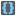• # The checker never finished checking my code

## Question related to missionBrackets

I would like to give some feedback about ...

So I finished writing my code, however when I press the run and check bottom the checker just keeps on running and never finish checking my code. Is there something wrong with my code or is it the checker's problem?

HTTP_USER_AGENT:

```Mozilla/5.0 (Windows NT 6.2; WOW64) AppleWebKit/537.36 (KHTML, like Gecko) Chrome/36.0.1985.125 Safari/537.36
```

My Code:

```def checkio(expression):
bracketExpression=""
for i in expression:
if i=="(" or i==")" or i=="{" or i=="}" or i=="[" or i=="]":
bracketExpression=bracketExpression+i
c=0
if len(bracketExpression)==0:
return True
else:
f=bracketExpression
empty=False
while not empty:
n=0
while n<len(f)-1:
if f[n]+f[n+1]=="()" or f[n]+f[n+1]=="[]" or f[n]+f[n+1]=="{}":
f=f[0:n]+f[n+2:]
n+=1
if len(f)==0:
return True
empty=True
else:
c+=1
if c==len(bracketExpression)/2:
return False
break;

#These "asserts" using only for self-checking and not necessary for auto-testing
if __name__ == '__main__':
assert checkio("((5+3)*2+1)") == True, "Simple"
assert checkio("{[(3+1)+2]+}") == True, "Different types"
assert checkio("(3+{1-1)}") == False, ") is alone inside {}"
assert checkio("[1+1]+(2*2)-{3/3}") == True, "Different operators"
assert checkio("(({[(((1)-2)+3)-3]/3}-3)") == False, "One is redundant"
assert checkio("2+3") == True, "No brackets, no problem"
```11